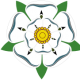# Levels

## Recommended PostsI have a number of drawings with levels on (single line and multitext) which are currently set to a local datum and I need to amend all to ordnance datum. To help reduce the time involved to do this I am looking for lisp routine that can find levels which are to 3 decimal places and add a set figure to it. (ie add 0.57m to every level on the drawing.

##### Share on other sitesHave a look on Lee Mac's site. I'm pretty sure he has a LISP program there that will do what you want.

##### Share on other sitesIf I understand your question, for similar situations, I use this routine. Unknown to the author.

```(defun chgterr (s)
(if (/= s "Function cancelled")
(princ (strcat "\nError: " s))
)
(setq p nil)
(setq *error* olderr)
(princ)
)

(defun C:INC (/ p l n e os as ns st s nsl osl sl si chf chm olderr)
(setq olderr  *error*
*error* chgterr
chm     0)
(setq p (ssget))
(setq inc (getreal "\nIncrement:"))
(setq l 0 n (sslength p))
(while (< l n)
(if (= "TEXT"
(cdr (assoc 0 (setq e (entget (ssname p l))))))
(progn
(setq s (cdr (setq as (assoc 1 e))))
(setq mn (atof s))
(setq nm (+ mn inc))
(setq s (rtos nm))
(setq e (subst (cons 1 s) as e))
(entmod e)
(setq chm (1+ chm))
)
)
(setq l (1+ l))
)
(princ chm)
(princ " text lines")
(princ " changed.")
(terpri)
(setq *error* olderr)
(princ)
)```

##### Share on other sitesPerfect that was exactly what I was looking for, Thanks for your help:)

##### Share on other sitesIf I understand your question, for similar situations, I use this routine. Unknown to the author.

```(defun chgterr (s)
(if (/= s "Function cancelled")
(princ (strcat "\nError: " s))
)
(setq p nil)
(setq *error* olderr)
(princ)
)

(defun C:INC (/ p l n e os as ns st s nsl osl sl si chf chm olderr)
(setq olderr  *error*
*error* chgterr
chm     0)
(setq p (ssget))
(setq inc (getreal "\nIncrement:"))
(setq l 0 n (sslength p))
(while (< l n)
(if (= "TEXT"
(cdr (assoc 0 (setq e (entget (ssname p l))))))
(progn
(setq s (cdr (setq as (assoc 1 e))))
(setq mn (atof s))
(setq nm (+ mn inc))
(setq s (rtos nm))
(setq e (subst (cons 1 s) as e))
(entmod e)
(setq chm (1+ chm))
)
)
(setq l (1+ l))
)
(princ chm)
(princ " text lines")
(princ " changed.")
(terpri)
(setq *error* olderr)
(princ)
)```

This is a bit simpler, and supports 'undo' functionality:

```(vl-load-com)

(defun c:FOO (/ *error* ss i acDoc)

(defun *error* (msg)
(if acDoc
(vla-endundomark acDoc)
)
(cond ((not msg))                                                   ; Normal exit
((member msg '("Function cancelled" "quit / exit abort")))    ; <esc> or (quit)
((princ (strcat "\n** Error: " msg " ** ")))                  ; Fatal error, display it
)
(princ)
)

(if (and (setq ss (ssget '((0 . "MTEXT,TEXT") (1 . "*#.#*"))))
(setq i (getreal "\nEnter increment: "))
)
(progn
(vla-startundomark
)
(vlax-for x (vla-get-activeselectionset acDoc)
(vla-put-textstring
x
(rtos (+ i (atof (vla-get-textstring x))) 2 3)
)
)
(prompt (strcat "\n** " (itoa (sslength ss)) " object(s) modified ** ")
)
)
)
(*error* nil)
)
```

##### Share on other sitesPerfect that was exactly what I was looking for, Thanks for your help:)

For TEXT and MTEXT:

```(defun chgterr (s)
(if (/= s "Function cancelled")
(princ (strcat "\nError: " s))
)
(setq p nil)
(setq *error* olderr)
(princ)
)

(defun C:INC (/ p l n e os as ns st s nsl osl sl si chf chm olderr)
(setq olderr  *error*
*error* chgterr
chm     0)
(setq p (ssget '((0 . "TEXT,MTEXT"))))
(setq inc (getreal "\nIncrement:"))
(setq l 0 n (sslength p))
(while (< l n)

(cdr (assoc 0 (setq e (entget (ssname p l)))))
(progn
(setq s (cdr (setq as (assoc 1 e))))
(setq mn (atof s))
(setq nm (+ mn inc))
(setq s (rtos nm))
(setq e (subst (cons 1 s) as e))
(entmod e)
(setq chm (1+ chm))
);progn
(setq l (1+ l))
); while

(princ chm)
(princ " text lines")
(princ " changed.")
(terpri)
(setq *error* olderr)
(princ)
);defun```

##### Share on other sitesFor TEXT and MTEXT:

```(defun chgterr (s)
(if (/= s "Function cancelled")
(princ (strcat "\nError: " s))
)
(setq p nil)
(setq *error* olderr)
(princ)
)

(defun C:INC (/ p l n e os as ns st s nsl osl sl si chf chm olderr)
(setq olderr  *error*
*error* chgterr
chm     0)
(setq p (ssget '((0 . "TEXT,MTEXT"))))
(setq inc (getreal "\nIncrement:"))
(setq l 0 n (sslength p))
(while (< l n)

(cdr (assoc 0 (setq e (entget (ssname p l)))))
(progn
(setq s (cdr (setq as (assoc 1 e))))
(setq mn (atof s))
(setq nm (+ mn inc))
(setq s (rtos nm))
(setq e (subst (cons 1 s) as e))
(entmod e)
(setq chm (1+ chm))
);progn
(setq l (1+ l))
); while

(princ chm)
(princ " text lines")
(princ " changed.")
(terpri)
(setq *error* olderr)
(princ)
);defun```

I sure hope the user always selects a valid selections set... Example: consider a TextString = "Bowties are cool".

## Join the conversation

You can post now and register later. If you have an account, sign in now to post with your account.
Note: Your post will require moderator approval before it will be visible.×   Pasted as rich text.   Paste as plain text instead

Only 75 emoji are allowed.